• 现有的很多函数库中所带的函数都只能进行一维数组计算，对于大量一维数组构成的二维数据只能通过循环进行迭代计算，在进行少量计算时，还能勉强使用，但一旦需要计算的量比较多时，每个量都需要进行循环计算，显得...
1.场景介绍
现有的很多函数库中所带的函数或自编函数都只考虑了最基本的一维数组的计算，对于大量一维数组构成的二维数据只能通过循环进行迭代计算，在进行少量计算时，还能勉强使用，但一旦需要计算的量比较多时（比如机器学习中大量特征的提取），每个量都需要进行循环计算，代码行上显得非常累赘，阅读性非常差。
2.拓展函数介绍
对于只有一个输入数据，其他输入均为参数的函数 ,例如：a,b,c...=f(x,para1,para2,para3,...)，可使用如下拓展函数Cal_by_coloumns将其拓展为可以进行二维计算的形式，由于使用的exec内置函数可以实现动态调用变量名，因此输出变量的个数不限。
具体用法是：
Cal_by_coloumns(Fun,x,**keys)
其中Fun代表需要拓展的一维计算函数，x为输入的数据（一维、二维均可），**keys为一个或多个函数的控制参数。 目前只支持输入一个数据变量x,对于需要输入多个数据变量的情况可根据需要对如下函数进行改造。所有输出将会相应拓展，若输入x的行数为N行（一维算作1行），则单个数值输出将变为N长数组，数组将变为行数为N的矩阵，以此类推。
其中的要点在于：1）使用收集参数，收集原函数中的参数；2）使用exec执行变量名可变情况下的循环计算。
# _*_coding: UTF-8_*_
# 开发作者 ：TXH
# 开发时间 ：2020-11-08 15:56
# 文件名称 ：Cal_by_coloumns.py
# 开发工具 ：Python 3.7 + Pycharm IDE
import numpy as np

def Cal_by_coloumns(Cal,x,**keys):
'''
将一维数组计算的Cal(x)函数，转换为按行计算的多维算法
:param Cal: 一维数组计算的函数名
:param x: Cal函数的输入，当前仅支持输入为一个变量的情况！
:return: 根据Cal的输出个数，输出对应长度的 list，每个元素对应一个输出变量
'''
if x.ndim == 1:
return Cal(x,**keys)
else:
if isinstance(Cal(x[0, :],**keys),tuple): # 输出为 tuple 说明有多个变量输出
L = len(Cal(x[0, :],**keys))
for i in range(L):
exec('y{}={}'.format(i, []))
for i in range(0, x.shape):
temp = Cal(x[i, :],**keys)
for j in range(L):
try:
exec('y{}.append({})'.format(j, temp[j]))
except:
exec('y{}.append({})'.format(j, list(temp[j]))) # 针对数组的情况
yy = []
for i in range(L):
exec('yy.append(np.array(y{}))'.format(i))
return yy
else: # 输出不为 tuple，说明只有一个变量输出
y = []
for i in range(0, x.shape):
y.append(Cal(x[i, :],**keys))
return np.array(y)

if __name__=='__main__':

# 以fft为例，将其拓展到2维按行计算，此处仅举例，实际根据需要对不同函数进行拓展
t=np.linspace(0,1,10000) # [1,1000] 线性插值得到1000个数，包含边界值
x=np.sin(2000*np.pi*t).reshape(2,-1)
F=Cal_by_coloumns(np.fft.fft,x,axis=-1)

# 绘图
import matplotlib.pyplot as plt
plt.plot(np.abs(F.T))


拓展函数可用在如下两种基本情况：
1）直接使用Cal_by_coloumns函数进行计算；见上述函数中带的例子。
2）将原来只能进行一维数组计算的数据封装拓展为支持二维计算的函数。拓展方法实例如下：
def normalize(x,method=1):
'''
按行进行标准化和归一化
:param x: 输入的待按行计算数据
:param method: 选定归一化方法:1.标准化（减均值除方差，标准化后范围为 -1.0 ~ 1.0） 2.归一化（归一化到 0.0 ~ 1.0）
:return: 输出结果
'''
def Cal(x,method): # 定义一个内部的只支持一维计算的函数
if method == 1:
return (x-x.mean())/x.var() # 标准化计算
else:
return (x - x.min()) / (x.max() - x.min()) # 归一化计算

return Cal_by_coloumns(Cal,x,method=method) # 将内部函数拓展为二维按行计算


展开全文python
• 将TXT文件中的数据读进二维数组，并对列数据进行线性插值再以txt文件将数据输出
• 类构造函数 bsarray.m 将 n 维数组作为输入，并计算 B 样条系数，用于内插或平滑任何小于或等于 7 的阶次的样条。 其他成员函数支持各种计算/操作： 间接过滤器.m：从存储在 bsarray 对象中的 BSpline 系数重建...matlab
• N维数组的快速最近邻插值(Nearest Neighbour)，Python实现 这份代码实现的是numpy.ndarray的快速最近邻插值（放缩）。而这个方法貌似并没有直接的API。 目标 我们由3*3的数组如下： [[1 2 3] [4 5 6] [7 8 9]] ...

这份代码实现的是numpy.ndarray的快速最近邻插值（放缩）。而这个方法貌似并没有直接的API（不能使用图像放缩API，因为数值会放缩到0-255）。

目标
我们由3*3的数组如下：
[[1 2 3]
[4 5 6]
[7 8 9]]

然后我们想要使用最近邻插值，放缩其成为一个8*10的矩阵，如下：
[[1 1 1 1 2 2 2 3 3 3]
[1 1 1 1 2 2 2 3 3 3]
[1 1 1 1 2 2 2 3 3 3]
[4 4 4 4 5 5 5 6 6 6]
[4 4 4 4 5 5 5 6 6 6]
[4 4 4 4 5 5 5 6 6 6]
[7 7 7 7 8 8 8 9 9 9]
[7 7 7 7 8 8 8 9 9 9]]

当前难点
显然我们可以使用相当简单的两重for循环实现，但是这种实现极其耗时，尤其是数组特别大的时候。  使用图像处理的API进行插值，会使得所有矩阵数值量化到0-255。  scipy.ndimage 库提供了一个API叫 zoom （在代码里面也提供了），但是会得到如下的结果，这显然不是我们想要的。
[[1 1 1 2 2 2 2 3 3 3]
[1 1 1 2 2 2 2 3 3 3]
[4 4 4 5 5 5 5 6 6 6]
[4 4 4 5 5 5 5 6 6 6]
[4 4 4 5 5 5 5 6 6 6]
[4 4 4 5 5 5 5 6 6 6]
[7 7 7 8 8 8 8 9 9 9]
[7 7 7 8 8 8 8 9 9 9]]

实现代码
ndarray_nearest_neighbour_scaling 为本文实现的算法ndarray_zoom_scaling 为使用 scipy.ndimage.zoom 实现的算法
import numpy as np
import scipy.ndimage

def ndarray_zoom_scaling(label, new_h, new_w):
"""
Implement scaling for ndarray with scipy.ndimage.zoom
:param label: [H, W] or [H, W, C]
:return: label_new: [new_h, new_w] or [new_h, new_w, C]
Examples
--------
ori_arr = np.array([[1, 2, 3],
[4, 5, 6],
[7, 8, 9]], dtype=np.int32)
new_arr = ndarray_zoom_scaling(ori_arr, new_h=8, new_w=10)
>> print(new_arr)
[[1 1 1 2 2 2 2 3 3 3]
[1 1 1 2 2 2 2 3 3 3]
[4 4 4 5 5 5 5 6 6 6]
[4 4 4 5 5 5 5 6 6 6]
[4 4 4 5 5 5 5 6 6 6]
[4 4 4 5 5 5 5 6 6 6]
[7 7 7 8 8 8 8 9 9 9]
[7 7 7 8 8 8 8 9 9 9]]
"""
scale_h = new_h / label.shape
scale_w = new_w / label.shape
if len(label.shape) == 2:
label_new = scipy.ndimage.zoom(label, zoom=[scale_h, scale_w], order=0)
else:
label_new = scipy.ndimage.zoom(label, zoom=[scale_h, scale_w, 1], order=0)
return label_new

def ndarray_nearest_neighbour_scaling(label, new_h, new_w):
"""
Implement nearest neighbour scaling for ndarray
:param label: [H, W] or [H, W, C]
:return: label_new: [new_h, new_w] or [new_h, new_w, C]
Examples
--------
ori_arr = np.array([[1, 2, 3],
[4, 5, 6],
[7, 8, 9]], dtype=np.int32)
new_arr = ndarray_nearest_neighbour_scaling(ori_arr, new_h=8, new_w=10)
>> print(new_arr)
[[1 1 1 1 2 2 2 3 3 3]
[1 1 1 1 2 2 2 3 3 3]
[1 1 1 1 2 2 2 3 3 3]
[4 4 4 4 5 5 5 6 6 6]
[4 4 4 4 5 5 5 6 6 6]
[4 4 4 4 5 5 5 6 6 6]
[7 7 7 7 8 8 8 9 9 9]
[7 7 7 7 8 8 8 9 9 9]]
"""
if len(label.shape) == 2:
label_new = np.zeros([new_h, new_w], dtype=label.dtype)
else:
label_new = np.zeros([new_h, new_w, label.shape], dtype=label.dtype)

scale_h = new_h / label.shape
scale_w = new_w / label.shape

y_pos = np.arange(new_h)
x_pos = np.arange(new_w)
y_pos = np.floor(y_pos / scale_h).astype(np.int32)
x_pos = np.floor(x_pos / scale_w).astype(np.int32)

y_pos = y_pos.reshape(y_pos.shape, 1)
y_pos = np.tile(y_pos, (1, new_w))
x_pos = np.tile(x_pos, (new_h, 1))
assert y_pos.shape == x_pos.shape

label_new[:, :] = label[y_pos[:, :], x_pos[:, :]]
return label_new

结果对比
比较了三个算法（两重for循环、scipy.ndimage.zoom 算法、本文实现的算法）在10,000次的插值操作后的总耗时，如下：
算法总耗时是我们方法耗时的倍数本文的方法0.360s/scipy.ndimage.zoom 算法0.436s1.21倍两重for循环1.523s4.23倍
显然本文的算法在速度上比两重for循环快很多。
展开全文Python
• 一维数组 // chapter07a02数组.cpp //熟练使用一位数组解决实际问题(标志符、数组元素、元素下标、元素类型) //掌握二维数组的使用 //使用数组实现常用算法 #include "pch.h" #include <iostream> using ...
一维数组
// chapter07a02数组.cpp
//熟练使用一位数组解决实际问题(标志符、数组元素、元素下标、元素类型)
//掌握二维数组的使用
//使用数组实现常用算法
#include "pch.h"
#include <iostream>
using namespace std;

int main()
{
const int N = 5;  //常量
int nums[N] = { 12,123,'C',123 };
cout << nums << endl;
int num1[] = { 12,123,true ,123 };
cout << num1 <<endl;
int days = {};
cout << days;
}

eg1:输入数组内容并打印
#include "pch.h"
#include <iostream>
using namespace std;

int main()
{
//求数组中的最大值和最小值,并输出对应下标
int nums[] = {8,54,65,84,52};
int numsLens = sizeof(nums) / sizeof(int); //计算出数组的长度
int max = nums;  //最大值
int min = nums;
int maxIndex = 0;  //最大值下标
int	minIndex = 0;

for (int i = 0; i < numsLens; i++)
{
if (max < nums[i])
{
max = nums[i];
maxIndex = i;
}
if (min > nums[i])
{
min = nums[i];
minIndex = i;
}
}
cout << "数组最大值为："<< max << " "<< "下标为：" << maxIndex << endl;
cout << "数组最小值为：" << min << " " << "下标为：" << minIndex << endl;
}

结果为：
数组最大值为：84 下标为：3
数组最小值为：8 下标为：0

eg2：冒泡排序：循环录入5个整型数字，进行降序排列后输出结果
// chapter08a01数组.cpp

#include "pch.h"
#include <iostream>
using namespace std;

int main()
{
//循环录入5个整型数字，进行降序排列后输出结果
//方案1：使用冒泡排序
// 第一轮比较的次数：数组的总长度-1
//下一轮比上一轮比较的次数少一次
int nums[] = {12,45,56,878,2};
int temp;
int numsLens = sizeof(nums) / sizeof(int); //计算出数组的长度
cout << "排序前的结果为：" ;
for (int i = 0; i < numsLens; i++)
{
cout << nums[i] << '\t';
}

//外层循环控制比较的轮数
for (int i = 0; i < numsLens -1 ; i++)
{
//内层循环控制每轮的比较和交换
for (int j = 0; j < 4 - i; j++)
{
if (nums[j] < nums[j + 1])
//交换
{
temp = nums[j];
nums[j] = nums[j + 1];
nums[j + 1] = temp;
}
}
}
cout << endl;
cout << "排序后的结果为：";
for (int i = 0; i < numsLens; i++)
{
cout << nums[i] << '\t';
}
}


eg3：选择排序：循环录入5个整型数字，进行降序排列后输出结果
#include "pch.h"
#include <iostream>
using namespace std;

int main()
{
//循环录入5个整型数字，进行降序排列后输出结果
//方案2：使用选择排序

int nums[] = {12,45,56,878,2};
int temp;  //临时变量
int numsLens = sizeof(nums) / sizeof(int); //计算整型数组的长度
cout << "排序前的结果为：" ;
for (int i = 0; i < numsLens; i++)
{
cout << nums[i] << '\t';
}
int min = nums;//假设最小值为数组里的第一个元素
int minIndex = 0;//最小值的初始下标为0

for (int i = 0; i < numsLens ; i++)
{
min = nums[i];
minIndex = i;

for (int j = i + 1; j < numsLens ; j++)
{
if (nums[j] < min)
{
min = nums[j];
minIndex = j;
}
}
//交换
if (minIndex > i)
{
temp = nums[minIndex];
nums[minIndex] = nums[i];
nums[i] = temp;
}
}

cout << endl;
cout << "排序后的结果为：";
for (int i = 0; i < numsLens; i++)
{
cout << nums[i] << '\t';
}

//逆序
for (int i = 0; i < numsLens / 2; i++)
{
temp = nums[i];
nums[i] = nums[numsLens - i - 1];
nums[numsLens - i - 1] = temp;
}
cout << endl;
cout << "逆序后的结果为：";
for (int i = 0; i < numsLens; i++)
{
cout << nums[i] << '\t';
}
}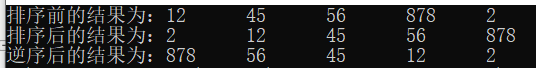eg4：数组的排序、插入和删除
#include "pch.h"
#include <iostream>
using namespace std;

int main()
{

//排序
double power;
int powerCount = 0; // 当前数组中的元素个数

power[powerCount++] = 71;
power[powerCount++] = 56;
power[powerCount++] = 41;
power[powerCount++] = 32;
power[powerCount++] = 14;

cout << "排序前的结果为：";
for (int i = 0; i < powerCount; i++)
{
cout << power[i] << '\t';
}
cout << endl;

//从大到小排序
double temp;
for (int i = 0; i < powerCount; i++)
{
for (int j = 0; j < powerCount - i - 1; j++)
{
if (power[j] < power[j + 1])
{
temp = power[j];
power[j] = power[j + 1];
power[j + 1] = temp;
}
}
}
cout << "排序后的结果为：";
for (int i = 0; i < powerCount; i++)
{
cout << power[i] << '\t';
}
cout << endl;

//插入元素（插入数值之后，仍是有序的）
double insertPower; //要插入的数值
int insertIndex = powerCount;  //默认插入到最后一个位置
cout << "请输入要插入的数值：";
cin >> insertPower;
//1.把新数值放在数组的末尾，重新进行排序
//1.找到第一个比插入数组大的位置 insertIndex
for (int i = 0; i < powerCount; i++)
{
if (insertPower > power[i])
{
insertIndex = i;
break;
}
}
//2.从最后一个元素开始，将数值复制到后一个元素中
for (int i = powerCount - 1; i >= insertIndex; i--)
{
power[i + 1] = power[i];
}

//3.将要插入的数字赋值给下标为insertIndex的元素
power[insertIndex] = insertPower;
//4.将数组的总长度加1
powerCount++;
cout << "插入后的结果为：";
for (int i = 0; i < powerCount; i++)
{
cout << power[i] << '\t';
}
cout << endl;

//删除元素
//1.找到要删除的目标
double deletePower;
int deleteIndex = -1;
cout << "请输入要删除的数值:";
cin >> deletePower;
for (int i = 0; i < powerCount; i++)
{
if (deletePower == power[i])
{
deleteIndex = i;
break;
}
}
if (deleteIndex == -1)
{
cout << "没有找到要删除的数字!" << endl;
}
else
{
//2.从找到的下标开始，后面的一个元素赋值给前面的一个元素
for (int i = deleteIndex; i < powerCount - 1; i++)
{
power[i] = power[i + 1];
}
//3.总长度 -1
powerCount--;
cout << "删除后的结果为：";
for (int i = 0; i < powerCount; i++)
{
cout << power[i] << '\t';
}
cout << endl;
}
}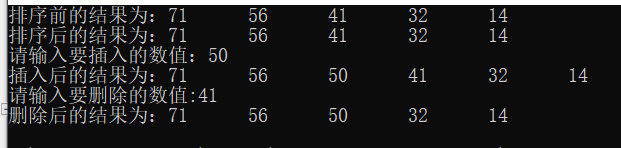展开全文c++ VS2017
• 做完之后，大师说这个程序还有优化的空间，因为计算机的内存时一块一维连续的空间，如果这里出发采用一维数组去实现，效果会更好，插2次值就OK，而且每次插值采用的时间复杂度为O(n)。同时涉及到一维数组及二维数组...
1.1前言
1.本文是博主本着虚心学习的态度与大家交流，博文难免会有错误之处，希望大家指正；  2.本文是针对对C语言以及图像放大的基础讨论，如是大牛们可以直接忽略本文；  3.运行环境：由于在不同电脑配置和系统上的运行时间有差异，本程序的测试平台：电脑CPU为Intel奔腾双核E6500，主频2.93GHZ内存4GB.虚拟机为10.0版的VMware，linux系统为Fedora16
要讲程序的运行速度，还是要从计算机的底层实现为出发点，以下一个图像的两倍线性放大论证此事实。刚刚接触图像处理，要完成图像的两倍线性放大，一想到图像有长与宽，最先想到用二维数组来实现，每次插值采用时间复杂度为O(n^2)的两个for循环来实现，插几次值就OK了。做完之后，大师说这个程序还有优化的空间，因为计算机的内存时一块一维连续的空间，如果这里出发采用一维数组去实现，效果会更好，插2次值就OK，而且每次插值采用的时间复杂度为O(n)。同时涉及到一维数组及二维数组的在堆的动态内存分配，以及以一位数组、二维数组作为函数的形参，进行参数传递。
主旨: 采用隔行隔列插入，左右及上下取均值把640x480的图像放大2倍，变成1280x960的图像，测试程序运行效率时选择了稍大点的数据，效果会比较明显。
2.1插值函数参数为二维数组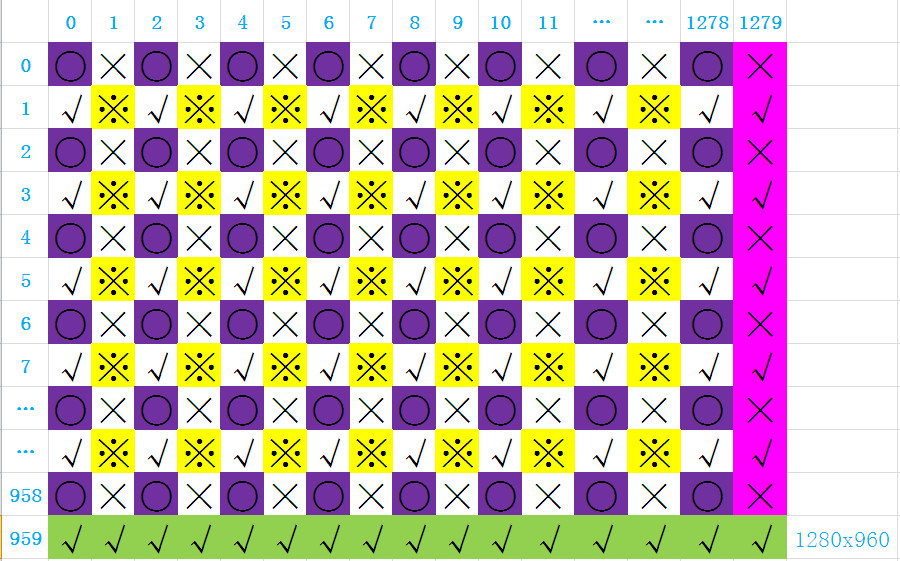紫色框的圆代表原始数据，首先上下取均值插(√)，再左右取均值插(×)，再取四个均值插(※)，最后插最后一列和最后一行。数据来源于计算机生成的伪随机数，为了方便测试该程序，改用较大分辨率2048x1536（现实没见到这种分辨率，按4:3的比例赋的值，测试用即可）程序从linux平台考过来的，格式可能会有一些乱。
源码如下：
#include <stdio.h>
#include <string.h>
#include <stdlib.h>
#include <ctype.h>
#include <sys/time.h>
typedef unsigned short dbyte;//图像每个像素点为双字节（双char型），且都大于零；char为8bit，short为16bit，用short来表示双char

#define H 1536  //表示图像分辨率的高度
#define W 2048  //表示图像分辨率的宽度

int interpolation_expansion(dbyte **original_data, dbyte **processed_data);//插值函数声明

int main(int argc, int *argv[])
{
int i, j;
dbyte **input_data, **output_data;
//内存分配
input_data = (dbyte **)malloc(H*sizeof(dbyte *));//为二维数组H行分配dbyte*内存
for(i = 0; i < H; i++)
{
input_data[i] = (dbyte *)malloc(W*sizeof(dbyte));//为每行中应放存放多少个dbyte元素分配内存
memset(input_data[i],0,W);//初始化内存
}
output_data = (dbyte **)malloc(2*H*sizeof(dbyte *));
for(i = 0; i < 2*H; i++)
{
output_data[i] = (dbyte *)malloc(2*W*sizeof(dbyte));
memset(output_data[i],0,2*W);
}
for(i = 0;i < H; i++)
{
for(j = 0; j < W; j++)
{
input_data[i][j] = rand();//数据采用伪随机数
}
}
for(i = 238; i < 241; i++)
{
for(j = 320; j < 324; j++)
printf("[%d][%d]_ %d  ",i , j, input_data[i][j]);
printf("\n");
}
struct timeval tpstart, tpend;
float timeuse;
gettimeofday(&tpstart, NULL);//获取函数调用之前的时间
interpolation_expansion(input_data, output_data);
gettimeofday(&tpend, NULL);//获取函数调用结束的时间
printf("_________________________<_________>_________________________\n");
for(i = 476; i < 481; i++)
{
for(j = 640; j < 648; j++)
printf("[%d][%d]_%d  ", i, j, output_data[i][j]);
printf("\n");
}
//计算函数调用多花费的时间，单位为秒
timeuse = 1000000*(tpend.tv_sec - tpstart.tv_sec)+      tpend.tv_usec - tpstart.tv_usec;
timeuse /= 1000000;
printf("The usetime TWO-dimensional array is %fS\n",timeuse);

//释放内存，【好像不用循环直接free(input_data)也可以，前提是需要知道第二维，我不是很确定】
for(i = 0; i < H; i++)
{
free(input_data[i]);
}
for(i = 0; i < 2*H; i++)
{
free(output_data[i]);
}
}

int interpolation_expansion(dbyte **original_data, dbyte **processed_data)//插值函数的原型
{
int i, j;
int last_line = 2*H -1;
int last_column = 2*W -1;
short tmp_data;

//set values for expansion array 把原来图片的像素点数据插入到需要放大的图片上（下标[偶数][偶数]标示○）
for(i = 0;i < H; i++)
{
for(j = 0; j < W; j++)
{
processed_data[2*i][2*j] = original_data[i][j];
}
}

//insert values into that subscript is odd line caculating by up number and down number用已知值进行上下取均值插入(下标[偶数][奇数]标示√)
for(i = 1; i < 2*(H-1); i += 2)//height_line
{
for(j = 0;j < 2*W; j += 2)//width_column
{

processed_data[i][j] =  (processed_data[i-1][j] +  processed_data[i+1][j])/2;
}
}

//insert values into that subscript is odd column caculating by left number and right number左右取均值插入(下标[奇][偶]标示×)
for(i = 0; i < 2*H; i += 2)//height_line
{
for(j = 1;j < 2*(W-1); j += 2)//width_column
{

processed_data[i][j] =  (processed_data[i][j-1] +  processed_data[i][j+1])/2;
}
}

//insert values into that both weight and height are odd numbers 对周围四个取均值（下标[奇][奇]标示※）
for(i = 1; i < 2*(H-1); i += 2)//height_line
{
for(j = 1; j < 2*(W-1); j += 2)
{
processed_data[i][j] = (processed_data[i-1][j-1] + processed_data[i-1][j+1] + processed_data[i+1][j-1] + processed_data[i+1][j+1])/4;

}
}
//insert values into the last line and column对最后一行和最后一列进行插值
for(i = 0; i< 2*H-1; i++)//insert values into last colum
{
if((tmp_data = 2*processed_data[i][last_column-1]-processed_data[i][last_column-2])>0)
{
processed_data[i][last_column] = tmp_data;
}
else
{
processed_data[i][last_column] = processed_data[i][last_column-1];
}
}
for(j = 0; j< 2*W-1; j++)//insert values into last line
{

if((tmp_data = 2*processed_data[last_line-1][j] - processed_data[last_line-2][j]) > 0)
{
processed_data[last_line][j] = tmp_data;
}else
{
processed_data[last_line][j] = processed_data[last_line-1][j];
}
}
processed_data[last_line][last_column] = (processed_data[last_line-1][last_column] + processed_data[last_line][last_column-1] + processed_data[last_line-1][last_column-1])/3;
return 1;
}
本段程序实现了函数形参为二维数组的传递，以及二维数组在堆的动态内存分配。我当初直接为二维数组在栈上分配空间，也就是指定死二维数组的行和列，但是当分辨率较高时，在linux编译能通过，但是运行遇到段错误（吐核），如下目前也没有找到其根本原因，后面用二维数组的动态内存分配解决了这个问题……
2.2程序的测试结果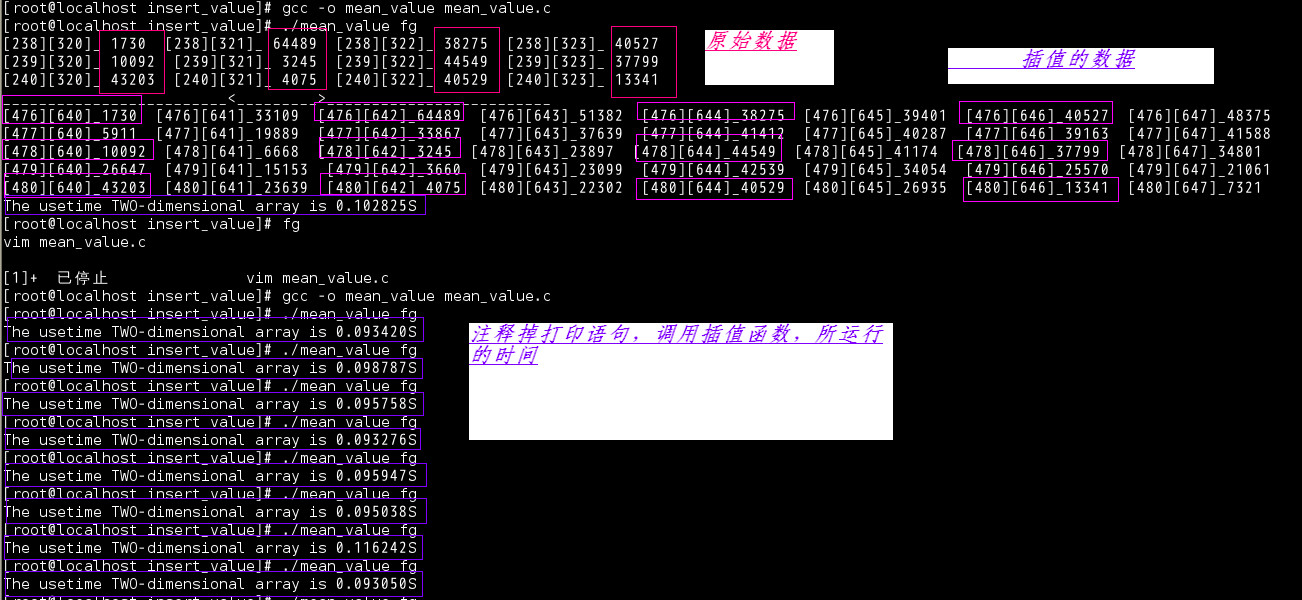截取的数据为放大前、后图片中间的某块数据，平均运行时间为0.098260秒
3.1插值函数参数为一维数组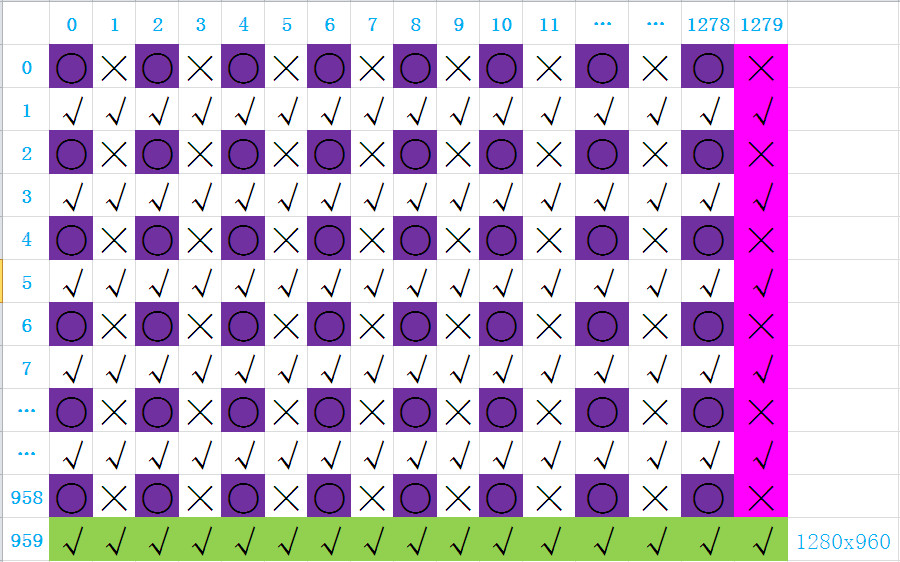紫色框的圆代表原始数据，2个for循环实现，首先左右取均值插值(×)，再上下取均值插值(√)；也可以在插值子函数里实现动态内存，但是为了方便管理，尽量在主函数分配，谁用谁分配和释放，只需要调用函数接口即可。
源码如下：
#include <stdio.h>
#include <string.h>
#include <stdlib.h>
#include <ctype.h>
#include <sys/time.h>

typedef unsigned short dbyte;
#define M 1536  //原始图片高度
#define N 2048  //原始图片宽度
#define H 3072    //height  放大2倍图片的高度
#define W 4096   //weight  放大2倍图片的宽度

dbyte* insert_values(dbyte *original_data, dbyte *processed_data);

int main(int argc, int *argv[])
{
int i, count;
//内存分配
dbyte *original_data = (dbyte *)malloc(M*N*sizeof(dbyte));
dbyte *processed_data = (dbyte *)malloc(W*H*sizeof(dbyte));
if(original_data == NULL)
{
printf("please allocate the input memery again!\n");
}

if(processed_data == NULL)
{
printf("please allocate the output memery again!\n");
}
//初始化内存为零
memset(original_data,0,M*N);
memset(processed_data,0,W*H);

//the test program as the follows.
//set original data
for(i  = 0; i < N*M; i++)
{
original_data[i] = rand();
}

//printf have not be to insert
for(i = 238*N + 320, count = 1; i < 324 + 241* N; )
{
printf(" %d__%ld   ",i, original_data[i]);
if(count%4 == 0)
{
count = 1;
i += (N-3);
printf("\n");
}else{
count++;
i++;
}
}
printf("______________*****************_____________________________*****************__________\n");

struct timeval tpstart, tpend;
float timeuse;
gettimeofday(&tpstart, NULL);
insert_values(original_data,processed_data);//use of the function
gettimeofday(&tpend, NULL);

//printf insert have been down
for(i = 476*W + 640, count = 1; i < 648 + 481* W; )
{
printf(" %d__%ld   ", i, processed_data[i]);
if(count%8 == 0)
{
count = 1;
i += (W-7);
printf("\n");
}else{
count++;
i++;
}
}
timeuse = 1000000*(tpend.tv_sec - tpstart.tv_sec)+ tpend.tv_usec - tpstart.tv_usec;
timeuse /= 1000000;
printf("The  usetime of One-dimensional array is %fS\n",timeuse);
free(processed_data);
free(original_data);
}

dbyte* insert_values(dbyte *original_data, dbyte *processed_data)//The value of return can be define by yourself
{
int i, j;
short tmp;
//set_values from original_data to the processed_data
for(i = j = 0; i<W*(H-1); i += 2, j++)//i为循环要放大的图像，步长为2（隔列插入），j用来循环遍历原始数据
{
if((i!= 0) && (i%W) == 0)//判断是否把一个W宽度的数据插完，真就跳W个数据继续插值，注意：如果不添加i!=0，当i=0，只判断i%W==0，最开始的W个数据时没有的
{
i += W;
}
processed_data[i] = original_data[j];
}

//insert_values into the line caculating by left number and right number 左右均值插值
for(i = 1;i < W*(H-1); i += 2)
{
if((i+1)%W == 0)//最后一列的插值方法，图像由左向右延伸
{
if((tmp = processed_data[i-1]*2-processed_data[i-2]) > 0) //如果中间值的2倍大于两边值之和，就插2倍中间值减去已知的值
{
processed_data[i] = tmp;
}
else{
processed_data[i] = processed_data[i-1];//如果需要插的值是小于零的，直接复制前一个像素点数据
}
i += W;
}
else
{
processed_data[i] = (processed_data[i-1] + processed_data[i+1])/2;

}
}

//insert_values into the line caculating by up number and down number
for(i = W; i < W*H; i++)
{
if(i < W*(H-1))
{
processed_data[i] = (processed_data[i-W] + processed_data[i+W])/2;
if((i+1)%W == 0)
{
i += W;
}
}else//最后一行的插值方法，图像由上向下延伸
{
if((tmp = processed_data[i-W]*2-processed_data[i-2*W]) > 0)
{
processed_data[i] = tmp;
}else{
processed_data[i] = processed_data[i-W];
}
}
}
return processed_data;
}
3.2一维数组程序的测试结果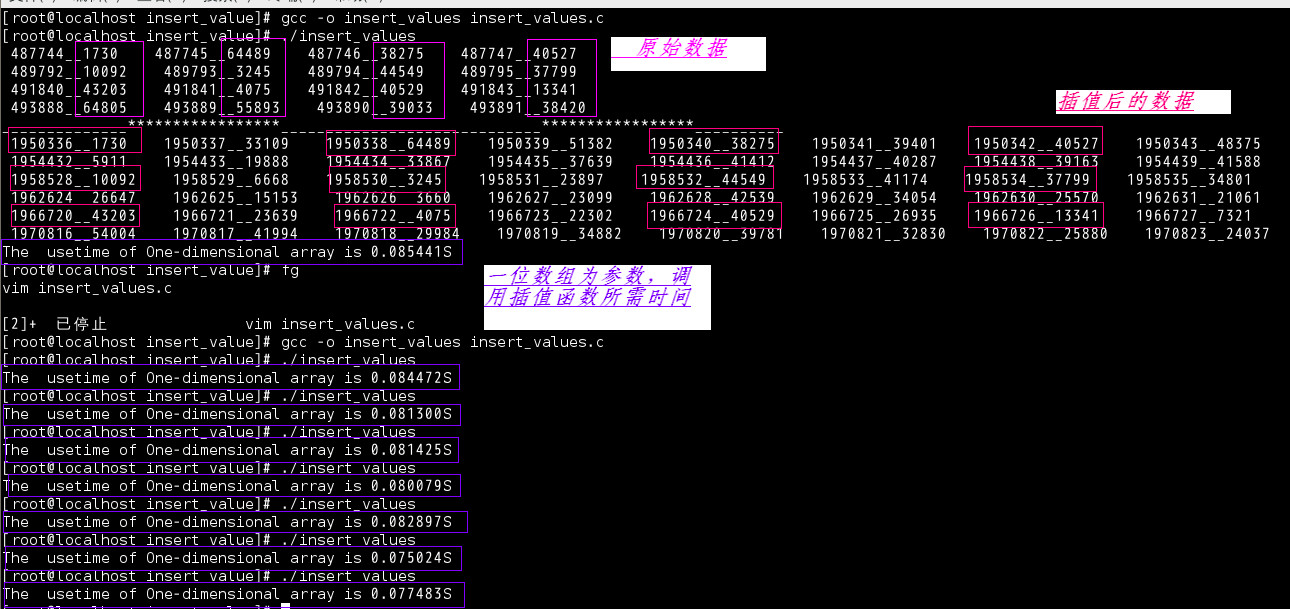截取的数据为放大前、后图片中间的某块数据，平均运行时间为0.081015秒
4.1结论
(0.098260-0.081015)=0.17245秒  0.17245/0.09826*100=17.55%,效率提高了百分之17.55。可能有的人觉得没有必要，毕竟现在的CPU处理速度已经相当快了。但如果是在嵌入式设备上就不一定了，毕竟嵌入式设备的资源都是有限的，所以很宝贵，充分利用它才是王道。
展开全文c语言 二维数组 动态内存分配
• matlab 二维插值计算matlab 二维插值计算matlab
• 定义个长度为5的整型数组； 定义两个float类型变量，用于保存总成绩、平均分，初始值为0； 定义两个int类型变量，用于保存最高分和最低分，初始值为0； 从控制台接收5名同学的成绩； 通过循环使数组的5个元素相加...java
• 文章目录、网格节点的插值计算二、散点数据的插值计算1. 示例 12. 示例 2 、网格节点的插值计算 Vq = interp2(X,Y,V,Xq,Yq) 使用线性插值返回双变量函数在特定查询点的插入值。结果始终穿过函数的原始采样。X 和...matlab 数学建模
• x – 表示将要计算插值点x坐标 xp – 表示已有的xp数组 fp – 表示对应于已有的xp数组的值 left – 表示当x值在xp中最小值左边时，x对应y的值为left right – 表示当x值在xp中最大值右边时，x对应y的值为right ...
• 尽管 numpy 自身提供了 numpy.interp 插值函数，但只能做一维线性插值，因此，在实际工作中，我们更多地使用 scipy 的 interpolate 子模块。关于 numpy 和 scipy 的关系，有兴趣的话，可以参考拙作《数学建模三剑客...numpy
• 2012 年 12 月 20 日 MATLAB 在二绘图中的简单应用(石磊 12011243923 2011级通信1班) [摘要]MATLAB提供了系列的绘图函数,用户不仅不许考虑绘图细节,只......(x) 画图: 画图:用 matlab 二绘图命令 plot ......
• x - 表示将要计算插值点x坐标 xp - 表示已有的xp数组 fp - 表示对应于已有的xp数组的值 left - 表示当x值在xp中最小值左边时，x对应y的值为left right -表示当x值在xp中最大值右边时，x对应y的值为ri...
• 众所周知，Global memory没有Cache，访问速度很慢，Shared memory访问速度很快，但是容量很小...在当前的CUDA版本中，3D的线性内存是无法直接绑定到texture memory，一维的可以，因此，需要将数据首先放进一个3D的CUDACUDA
• 先来看段代码：#include <stdio.h> #define SIZE (4)void PrintMatrix(int **arr) { for (int i = 0; i < SIZE; i++) { for (int j = 0; j < SIZE; j++) { printf(" %d", arr[i][j]); }C C语言 C++
•griddata python
• MATLAB 提供了 interp1(x,y,xq,'Method')函数命令可以进行一维插值，其中一维插值有四种常用的方法，也就是 ‘Method’ 可以选择邻近点插值Nearest，线性插值Linear，三次样条插值Spline和立方插值Pchip。...matlab 数学建模
•  1、yi=interp1(x,Y,xi):返回插值向量yi，每个元素对应于参量xi，同时由向量x与Y，的内插值决定。  参量x指定数据Y的点。若Y为矩阵，则Y按每列计算，yi的阶数是 length(xi)*size(Y,2)  2、yi=interp1（x,Y,...
• ## MATLAB 一维插值

千次阅读 2014-09-29 20:14:18
MATLAB 插值   常用的多项式插值法： 1.拉格朗日插值 拉格朗日插值插值的基本公式，由n+1个n次插值基函数构成，即n次插值多项式。 一般：matlab
• 1, 一维插值interp1(x,y,X1,method) x = linspace(0,10,11) y = sin(x) plot(x,y,'-ro') 插值方法有如下： method=‘nearest’,‘linear’,‘spline’,‘pchip’,‘cubic’ 比如使用三次条样插值spline，则 x = ...
• 一维插值 插值不同于拟合。插值函数经过样本点，拟合函数一般基于最小二乘法尽量靠近所有样本点穿过。常见插值方法有拉格朗日插值法、分段插值法、样条插值法。 拉格朗日插值多项式：当节点数n较大时，拉格朗日插值...
• excel、二、三维插值 来吧，给个好评吧，谢谢......
• 一维插值 常规用法 【参考文章】 python实现各种插值法(数值分析) python scipy样条插值函数大全（interpolate里interpld函数） Python：插值interpolate模块 示例代码 # -*-coding:utf-8 -*- import numpy as np ...python
• 目录前言MATLAB插值函数三绘图绘制立体曲线图绘制曲面图等高线在XY平面的投影 前言 调用一下MATLAB自带的seamount.mat数据文件 load seamount plot3(x,y,z,'.','markersize',12) xlabel('Longitude'), ylabel('...matlab
• 从已知的一些离散数据点及其函数值，即函数的列表法表示，推求出未知点上函数值的所谓插值方法，在科技工作中应用十分广泛，如查对数表、三解函数表中都会遇到这类插值问题。MATLAB中设有许多插值命令，以下介绍最...
• 关于如何使用scipy进行一位和二维插值官方文档介绍的已经非常详细，基本上根据demo操作就能搞清楚怎么使用scipy进行一维和二维插值。但是有时发现自己需要使用scipy进行三维和更高维插值，然而官方文档对于如何进行...scipy python 算法
• NumPy(Numerical Python)是 Python 语言的个扩展程序库。其中提供了许多向量和矩阵...拥有个类似于列表的、强大的N维数组对象ndarray，它描述了相同类型的元素的集合。具有实用的线性代数、傅里叶变换和随机生成python numpy...